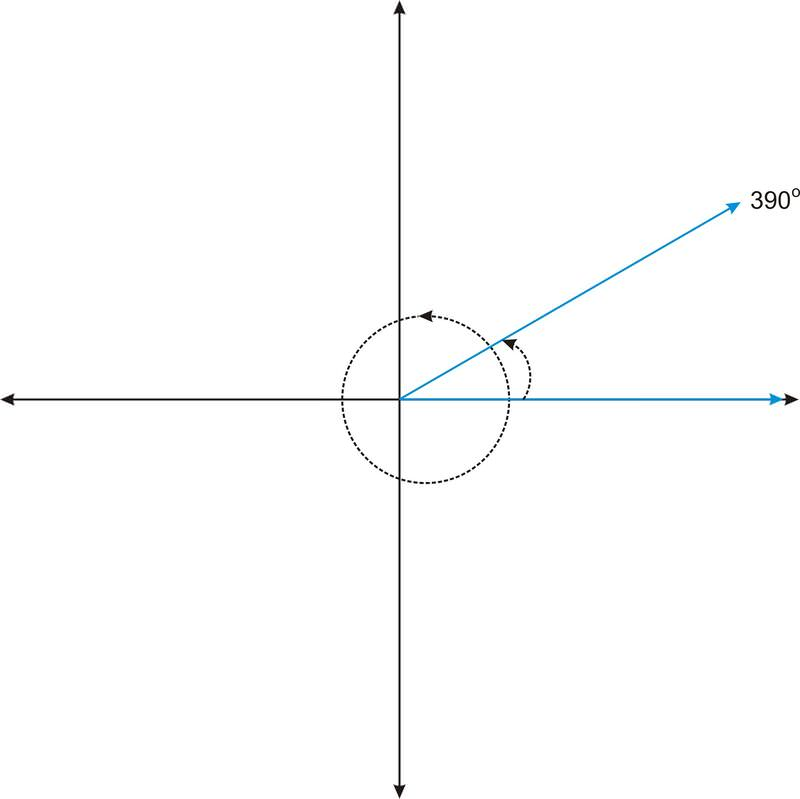Skip to main content

# 2.3.4: Coterminal Angles

•• Contributed by CK12
• CK12

Set of angles with the same terminal or end side.

While playing a game with friends, you use a spinner that looks like this:Figure $$\PageIndex{1}$$

As you can see, the angle that the spinner makes with the horizontal is $$60^{\circ}$$. Is it possible to represent the angle any other way?

### Coterminal Angles

Consider the angle $$30^{\circ}$$, in standard position.

Now consider the angle $$390^{\circ}$$. We can think of this angle as a full rotation ($$360^{\circ}$$), plus an additional 30 degrees.Figure $$\PageIndex{3}$$

Notice that $$390^{\circ}$$ looks the same as $$30^{\circ}$$. Formally, we say that the angles share the same terminal side. Therefore we call the angles co-terminal. Not only are these two angles co-terminal, but there are infinitely many angles that are co-terminal with these two angles. For example, if we rotate another $$360^{\circ}$$, we get the angle $$750^{\circ}$$. Or, if we create the angle in the negative direction (clockwise), we get the angle $$−330^{\circ}$$. Because we can rotate in either direction, and we can rotate as many times as we want, we can continuously generate angles that are co-terminal with $$30^{\circ}$$.

#### Identifying Co-Terminal Angles

For the following questions, determine if the angle is co-terminal with $$45^{\circ}$$.

1. $$−45^{\circ}$$

No, it is not co-terminal with $$45^{\circ}$$

2. $$405^{\circ}$$

Yes, $$405^{\circ}$$ is co-terminal with $$45^{\circ}$$.

3. $$−315^{\circ}$$

Yes, $$−315^{\circ}$$ is co-terminal with $$45^{\circ}$$.

Example $$\PageIndex{1}$$

Earlier, you were asked if it is possible to represent the angle any other way.

Solution

You can either think of $$60^{\circ}$$ as $$420^{\circ}$$ if you rotate all the way around the circle once and continue the rotation to where the spinner has stopped, or as $$−300^{\circ}$$ if you rotate clockwise around the circle instead of counterclockwise to where the spinner has stopped.

Example $$\PageIndex{2}$$

Find a coterminal angle to $$23^{\circ}$$

Solution

A coterminal angle would be an angle that is at the same terminal place as $$23^{\circ}$$ but has a different value. In this case, $$−337^{\circ}$$ is a coterminal angle.

Example $$\PageIndex{3}$$

Find a coterminal angle to $$−90^{\circ}$$

Solution

A coterminal angle would be an angle that is at the same terminal place as −90^{\circ}\) but has a different value. In this case, $$270^{\circ}$$ is a coterminal angle.

Example $$\PageIndex{4}$$

Find two coterminal angles to $$70^{\circ}$$ by rotating in the positive direction around the circle.

Solution

Rotating once around the circle gives a coterminal angle of $$430^{\circ}$$. Rotating again around the circle gives a coterminal angle of $$790^{\circ}$$.

### Review

1. Is $$315^{\circ}$$ co-terminal with $$−45^{\circ}$$?
2. Is $$90^{\circ}$$ co-terminal with $$−90^{\circ}$$?
3. Is $$350^{\circ}$$ co-terminal with $$−370^{\circ}$$?
4. Is $$15^{\circ}$$ co-terminal with $$1095^{\circ}$$?
5. Is $$85^{\circ}$$ co-terminal with $$1880^{\circ}$$?

For each diagram, name the angle in 3 ways. At least one way should use negative degrees.

1.Figure $$\PageIndex{4}$$
2.Figure $$\PageIndex{5}$$
3.Figure $$\PageIndex{6}$$
4.Figure $$\PageIndex{7}$$
5.Figure $$\PageIndex{8}$$
6. Name the angle of the 8 on a standard clock two different ways.
7. Name the angle of the 11 on a standard clock two different ways.
8. Name the angle of the 4 on a standard clock two different ways.
9. Explain how to determine whether or not two angles are co-terminal.
10. How many rotations is $$4680^{\circ}$$?

### Review (Answers)

To see the Review answers, open this PDF file and look for section 1.16.

## Vocabulary

Term Definition
Coterminal Angles A set of coterminal angles are angles with the same terminal side but expressed differently, such as a different number of complete rotations around the unit circle or angles being expressed as positive versus negative angle measurements.

## Additional Resources

Interactive Element

Practice: Coterminal Angles

• Was this article helpful?